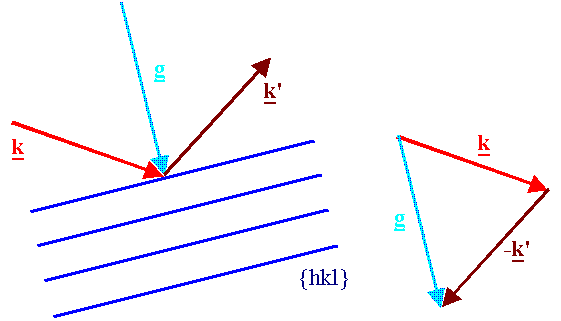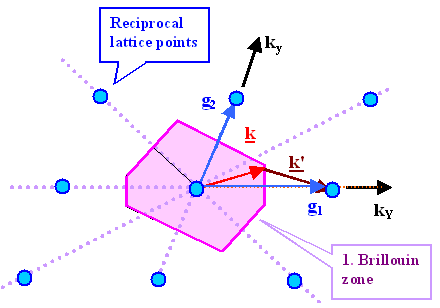### 2.1.2 Diffraction of Electron WavesNote: It is too tiresome to underline all vectors, and we will simply stop doing it except if it is absolutely necessary.Electron waves like all waves experience diffraction effects in periodic structures like crystals. This is best described in the reciprocal lattice of the crystal in question. There are several ways to construct a reciprocal lattice from a space lattice.Remember that a lattice – in contrast to a crystal – is a mathematical construct. A lattice becomes becomes a crystal by putting a set of atoms – the base of the crystal – at every lattice point.There are 14 different kinds of lattices – the Bravais lattices – with different symmetries that are sufficient to describe the lattice of any crystal.If you are unsure about this topic, refer to the appropriate chapter in "Introduction to Materials Science I" (in German) or in "Defects" (in English)Lets look at three definitions of the reciprocal lattice, which are – of course – all equivalent but at different levels of abstraction. This is elaborated in the link.1. The reciprocal lattice is the Fourier transform of the space lattice.2. The reciprocal lattice with an elementary cell (EC) as defined by the base vectors b1,2,3 is obtained from the space lattice as defined by its base vectors a1,2,3 by the equations

 a2× a3 ax × (ay · az ) b1 = 2p
 a3× a1 ax × (ay · az ) b2 = 2p
 a1× a2 ax × (ay · az ) b3 = 2p

ax × (ay · az )  =  volume V of EC3. The base vectors of the reciprocal lattice can be constructed by drawing vectors perpendicular to the three {100} planes of the space lattice and taking their length as 2p/dhkl with dhkl = spacing between the lattice planes with Miller indices {hkl}.You will, of course, never confuse the spacing between lattice planes with the spacing between crystal planes, i.e. sheets of atoms, which may be something different.

Bragg ConditionIf a wave impinges on a crystal – and it doesn't matter if it is an electromagnetic wave, e.g. X-rays, or an electron, or neutron "wave" – it will be reflected at a particular set of lattice planes {hkl} characterized by its reciprocal lattice vector g only if the so-called Bragg condition is met.Let the wave vector of the incoming wave be k, the wave vector of the reflected wave is k'. The Bragg condition correlates the three vectors involved – k, k', and g – in the simplest possible form:
k  –  k'  =  gThere is no simpler relation correlating three vectors – mother nature again makes life as easy as possible for us!This Bragg condition is easily visualized:If, and only if the three vectors involved form a closed triangle, is the Bragg condition met. If the Bragg condition is not met, the incoming wave just moves through the lattice and emerges on the other side of the crystal (neglecting absorption).So far we assumed implicitly that the diffraction (or, in more general terms, the scattering) of the incoming wave is elastic, i.e. the magnitude of k' is identical to that of k, i.e. |k| = |k'| – which means that the diffracted wave has the same momentum, wavelength and especially energy as the incoming wave.This is not necessarily implied by Bragg's law – the equation kk' = g can also be met if k' differs from k by a reciprocal lattice vector; i.e. if scattering with a change of energy takes place. This will become important later.

Brillouin ZonesIn X-ray analysis of crystals we often deal with an (idealized) situation were one well-defined plane wave with fixed wavelength impinges on a crystal, and the question is which set of lattice planes will reflect the one incoming beam.This question can be answered most easily in a qualitative way by a geometric construction called the Ewald construction which can be found in the "Introduction to Materials Science II" Hyperscript.However, if we consider the diffraction effects occurring to the free electrons contained in the crystal, we are looking at a (quasi) continuum of wave vectors: We have all possible directions and many wavelengths.The question is now a bit different: We want to know which particular wave vectors out of many (an infinite set, in fact) meet the Bragg condition for a given crystal lattice plane.This question can be answered most easily and semi-quantitatively by a geometric construction called the Brillouin construction. Lets look at it in a simplified two-dimensional form.If we construct WignerSeitz cells in the reciprocal lattice as shown by the pink lines, all wave vectors ending on the Wigner-Seitz cell walls will meet the Bragg condition for the set of lattice planes represented by the cell wall.The Wigner–Seitz cells form a nested system of polyhedra which can be numbered according to size. These cells are called Brillouin zones (BZ); the smallest one is called the 1. BZ, the next smallest one the 2. BZ, and so on.All wave vectors that end on a BZ wall will fulfill the Bragg condition and thus are diffracted. Of course, the origin must be at the center of that BZ.Wave vectors completely in the interior of the 1. BZ, or in between any two BZs, will never get diffracted; they move pretty much as if the potential would be constant, i.e. they behave very close to the solutions of the free electron gas.Of course, if we talk about diffraction in a crystal, we assumed implicitly that the potential is no longer constant, but periodic with the crystal.The statement above is thus not trivial, but a first important conclusion from diffraction geometry alone: We have good reasons (albeit no ironclad justification) to believe that the free electron gas model is a decent approximation for electrons with wave vectors not ending on (or close to) a Brillouin zone edge of the crystal in question.We thus only have to consider what happens to the electrons with wave vectors on or close to a BZ edge. In which properties do they differ from electrons of the free electron gas model?
 Questions Quick Questions to 2.1.2

© H. Föll (Semiconductors - Script)